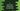# C program to read user input string and print each word in a new line## Introduction :

In this C programming tutorial, we will learn how to read one user input string and how to print each word of that string in a newline.

Our program will ask the user to enter a string. It will read that string and print each word of that string in a new line.

For example, if the input string is Hello World !!, it will print the below output :

``````Hello
World
!!``````

## C program :

``````#include <stdio.h>
#include <string.h>

// 1
#define MAX_SIZE 100

int main()
{
// 2
char str[MAX_SIZE];

// 3
printf("Enter a string : ");
fgets(str, MAX_SIZE, stdin);

int i;
int end;
int start = 0;

// 4
for (i = 0; i < strlen(str); i++)
{
// 5
if (str[i] == ' ' || i == strlen(str) - 1)
{
end = i;
printf("%.*s\n", (end - start), str + start);
start = i + 1;
}
}

return 0;
}``````

## Explanation :

The commented numbers in the above program denote the step numbers below :

1. MAX_SIZE is defined as 100. It is the maximum size of a string that this program can read.
2. str is a character array of size MAX_SIZE. We will store the user input string in this array.
3. Ask the user to enter a string. Read that string and store it in the str variable by using the fgets method. Next, we are defining three variables: i to use in a loop, start to store the current start index of the current word, and end to store the current end index of the current word.
4. Run one for loop to iterate through the characters of the user input string.
5. If the current loop iteration gets any blank space or end of the string, it means that the end of a word is reached. So, it will print that word starting from the start index to the end index in the string for that word. start and end are used to indicate the start index and end index of the current word.

### Sample Output :

``````Enter a string : hello world !!
hello
world
!!

Enter a string : the quick brown fox jumps over the dog
the
quick
brown
fox
jumps
over
the
dog``````